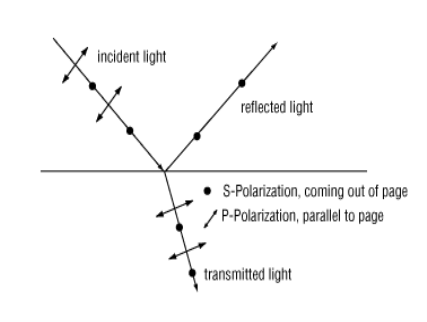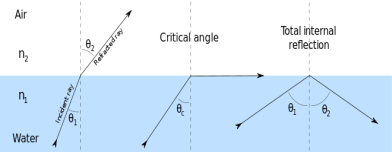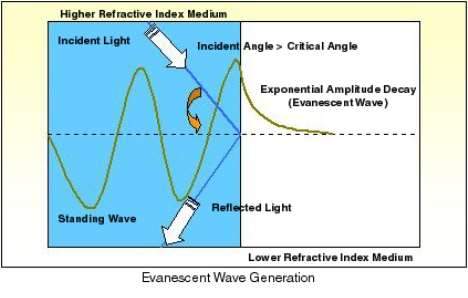Disclaimer: This is an example of a student written essay.

Any scientific information contained within this essay should not be treated as fact, this content is to be used for educational purposes only and may contain factual inaccuracies or be out of date.

# Sensing of Human Blood Group by SPR Based Sensors

 ✅ Paper Type: Free Essay ✅ Subject: Physics ✅ Wordcount: 3257 words ✅ Published: 12th Mar 2018

Contents-

• Introduction

Basic of Surface Plasmon

P and S polarized light waves

Properties of Surface Plasmon Waves

Transverse Electric and Transverse Magnetic Waves

Dispersion Relation for Surface Plasmon

• Principle

Total Internal Reflection

N-Layer Model and Fresnel’s Relation

Evanescent Waves

Resonance Condition

Minimum of Reflectance at Resonance

Angular Interrogation and Wavelength Interrogation

Performance parameters for SPR based sensors

• Experiment

Kretscheman and Otto Configuration

Coating of metal layer by Vacuum Coating Machine

Basic Of Surface Plasmons-

The electron charges on a metal boundary can perform coherent fluctuations hence collective charge density oscillations of electrons at metal-dielectric interface are known as plasma oscillations and the quantum of these oscillations are known as surface plasmons. The surface plasmons are accompanied by a longitudinal (TM- or P-polarized) electric field which decays exponentially in metal as well as in dielectric medium. The electric field has its maximum at metal-dielectric interface. The TM- polarization and exponential decay of electric field are found by solving the Maxwell equation. The charge motion in a surface plasmon always creates electromagnetic fields outside (as well as inside) the metal. The total excitation, including both the charge motion and associated electromagnetic field, is called either surface plasmon polaritonat a planar interface. .

Properties of Surface Plasmon Waves-

Surface Plasmon waves are the surface localized electromagnetic waves produced by the collective resonating oscillations of free electrons on the plasma surface. The surface Plasmon is a transverse wave propagating along the plasma surface with the oscillating electric field vector normal to the surface. The two main properties of the surface Plasmon waves are firstly they are of exponentially decaying nature, when propagate along the surface of metal-dielecric interface it gets decay both in metal as well as in dielectric and secondly it gets excited only by P-polarized incident light wave.

P-polarized and S-polarized light waves-When the electric field vector of incident light wave is perpendicular to the plane of incidence than light wave is called S-polarized light wave and when the electric field vector are parallel to plane of incidence than light wave is called P-polarized light wave.

Transverse Electric and Transverse Magnetic Waves

If the incident, transmitted and reflected waves all have a wave vector component along the z direction, that is, they have an effective velocity along z. The fields Ei,perp, Er,perp, and Et,perp are all perpendicular to the z direction. These waves are called transverse electric field (TE) waves. On the other hand, waves with Ei,//, Er,//, and Et,// only have their magnetic field components perpendicular to the z direction and these are called transverse magnetic field (TM) waves.

Dispersion Relation for Surface Plasmon-

• Principle

Total internal reflection-

Internal Reflectionis a phenomenon that happens when a propagatingwave strikes a medium boundary at an angle larger than a particular critical anglewith respect to thenormalto the surface. If the refractive indexis lower on the other side of the boundary and the incident angle is greater than the critical angle, the wave cannot pass through and is entirelyreflected. The critical angleis theangle of incidenceabove which the total internal reflectance occurs. This is particularly common as anoptical phenomenon, where light waves are involved, but it occurs with many types of waves, such aselectromagnetic wavesin general orsound waves. When a wave crosses a boundary between materials with different kinds of refractive indices, the wave will be partiallyrefractedat the boundary surface, and partially reflected. However, if the angle of incidence is greater (i.e. the direction of propagation or ray is closer to being parallel to the boundary) than the critical angle – the angle of incidence at which light is refracted such that it travels along the boundary – then the wave will not cross the boundary and instead be totally reflected back internally. This can only occur when the wave in a medium with a higher refractive index (n1) hits its surface that’s in contact with a medium of lower refractive index (n2). For example, it will occur with light hittingairfrom glass, but not when hitting glass from air.Fresnel’s Relation

We will describe the incident, reflected and refracted waves by the exponential representation of a travelling wave, i.e.,

Where r is theposition vector, the wavevectors ki, kr, kt describe respectively the derictions of the incident, reflected and transmitted waves and Eio, Ero and Eto are the respective amplitudes. Any phase changes such as phir and phit in the reflected and transmitted waves with respect to the phase of the incident wave are incorporated into the complex amplitudes Ero and Eto. Our objective is to find Ero and Eto with respect to Eio.

If you need assistance with writing your essay, our professional essay writing service is here to help!

Similar equations can be stated for the magnetic field components in the incident, reflected and transmitted waves but will be perpendicular to the corresponding electric fields. The electric and magnetic fields anywhere on the wave must be perpendicular to each other as a requirement of electromagnetic wave theary. This means that with E// in the EM wave we have a magnetic field Bperp associated with it such that Bperp= (n/c)E//. Simillarly, Eperp will have a magnetic field B// associated with it such that B//=(n/c)Eperp.

There are two useful fundamental rules in electromagnetism that govern the behaviour of the electric and magnetic fields at a boundary between two dielectric media which we can arbitrary label as 1 and 2. These rules are called boundary conditions. The first states that the electric field that is tangential to the boundary surface Etangential must be continuous across the boundary from medium 1 to 2 i.e., at the boundary y=0.

Etangential(1)=Etangential(2)

The second rule is that the tangential component of the magnetic field Btangential to boundary must be likewise continuous from medium 1 to 2 provided that the two media are non-magnetic (relative permeability µr=1),

Btangential(1)=Btangential(2)

Using these boundary conditions for the fields at y=0, and the relationship between the electric and magnetic fields, we can find the reflected and transmitted waves in terms of the incident wave. The boundary conditions can only be satisfied if the reflection and incidence angles are equal, @r=@i, and the angles for the transmited and incident waves obey Snell’s n1sin@i=n2sin@r.

Applying the boundary conditions to the EM wave going from medium 1 to medium 2, the amplitudes of the reflected and transmitted waves can be readily obtained in terms of n1, n2, and incidence angle @i alone. These relationships are called Fresnel’s equations. If we define n=n2/n1, as the relative refractive index of medium 2 to that of 1, then the reflection and transmission coefficients for Eperp are

Rperp

And

Tperp

These are corresponding coefficients for the E// fields with corresponding reflection and transmission coefficients r// and t//:

r//

t//

Further, the reflection and transmission coefficients are related by

r// + nt// = 1 rperp +1 = tperp

The significance of these equations is that they allow the amplitudes and phases of the reflected and transmitted waves to be determined from the coefficients rperp, r//, t//, and tperp.

Evanescent waves-

Evanescent waves are formed when sinusoidal waves are (internally) reflected off an interface at an angle greater than the critical angle so that total internal reflection occurs. “Evanescent” means “tending to vanish”, which is appropriate because the intensity of evanescent waves decays exponentially with distance from the interface at which they are formed. Any propagating wave is converted into an evanescent wave when hitting a classically forbidden region. In this case, at least one component of the wave vector kbecomes imaginary or complex and the wave experiences exponential damping when propagating in this region.In the case of an optical fibre the cladding has less refractive index than the core of the fibre. When light experience total internal reflection at the core-cladding interface, some of the energy of the light waves in the core of the fibre penetrate into the cladding for a very short distance. The energy flow of this evanescent wave is parallel to the surface of the core and in the same direction as the main flow of energy within the core.

Resonance Condition-

The propagation constant (Ksp)

Of the surface Plasmon wave propagating along the metal-dielectric interface is given by-

Where εm and εs are the dielectric constants of metal and the dielectric medium respectively,

ω is the frequency of incident light, and

c is the velocity of light

and from above equation it may be noted that the propagation constant of surface Plasmon wave depends on the dielectric constants of both the metal and the dielectric medium.

The surface plasmons can be excited by light with same polarization state as that of surface Plasmons. The propagation constant (Ks) of the light wave with frequency w propagating through the dielectric medium is given by-

Since εm< 0 (for metal) and εs>0 (for dielectric), for a given frequency, the propagation constant of surface Plasmon (Ksp) is greater than that of light wave in dielectric medium (Ks). To excite surface plasmons, two propagation wave- vectors should be equal. Hence, the direct light cannot excite surface plasmons at a metal-dielectric interface. To excite surface plasmons their momentum and hence the wave vector of the exciting light in dielectric medium should be increased. This can be done if instead of a direct light, evanescent wave is used to excite the surface plasmons. To obtain the evanescent wave for the excitation of surface plasmons, a prism with high dielectric constant is used.

Our academic experts are ready and waiting to assist with any writing project you may have. From simple essay plans, through to full dissertations, you can guarantee we have a service perfectly matched to your needs.

When a light beam is incident through one of the two sides of the prism at an angle greater than the critical angle at prism-air interface the total internal reflection of light beam takes place. In the condition of total internal reflection light beam does not return exactly from the interface. Instead it returns after penetrating in the lower refractive index medium (air in this case). The field in the lower refractive index medium is called evanescent field and the wave corresponding to this is called evanescent wave. The evanescent wave propagates along the prism-air interface and decays exponentially in the rarer medium (air). The propagation constant of the evanescent wave at prism-air interface is given by-

Where,

εp, represents the dielectric constant of the material of the prism and

θ is the angle of incidence of the beam.

Increase in the dielectric constant of the prism increases the propagation constant of the evanescent wave and hence this can be made equal to propagation constant of the surface Plasmon wave to propagation constant of the surface Plasmon wave to satisfy the surface Plasmon resonance condition.

When a p-polarized light beam is incident through the prism on the prism-metal layer interface at an angle θ equal to or greater than the critical angle, the evanescent wave is produced at the prism-metal interface. The excitation of surface plasmons occurs when the wave vector of evanescent wave exactly matches with that of the surface plasmons of similar frequency. This occurs at a particular angle of incidence θres. Thus the resonance condition for surface Plasmon resonance is-

The excitation of surface plasmons at metal-dielectric interface results in the transfer of energy from incident light to surface plasmons, which reduces the intensity of the reflected light. If the intensity of the reflected light is measured as a function of angle of incidence θ for a fixed values of frequency, metal layer thickness and dielectric layer thickness then a sharp dip is observed at resonance angle, θres, due to an efficient transfer of energy to surface plasmons.

Minimum of Reflectance at Resonance

The excitation of surface plasmons at metal-dielectric interface results in the transfer of energy from incident photons to surface plasmons, which reduces the energy of the reflected light. If the normalized reflected intensity (R), which is basically the output signal, is measured as a function of incident angle @ by keeping other parameters and components (such as frequency, metal layer, and dielectric layer) unchanged, then a sharp dip is observed at resonance angle @sp due to an efficient transfer of energy to surface plasmons

The minimum of the normalized reflected intensity (R) can be quantitatively described with the help of Fresnel’s equations for the three-layer system p/m/s. Here,

p, stands for high refractive index material prism

m, stands for metal film of thickness dm and

s, stands for low index dielectric medium, eg: air, water etc.

The light wave is incident at an angle greater than the corresponding ATR angle. At this point, one important fact is the energy conservation that requires R+A+T=1, that is, the sum of relative reflection, absorption, and transmission is unity. Since, T=0 at ATR, hence we are left with A+R=1 in the present case.

The light wave having passed the glass prism (εp), is reflected partially at prism interface. A part of the incident light wave energy traverses the metal film(of thickness dm) as an exponential decaying evanescent wave. At the metal-dielectric (m/s) interface, it induces the surface Plasmon excitation, which radiate light back into the metal film. If the metal layer thickness (dm) is small, the back scattered field tends to increase. Since, this back scattered wave is out-of-phase with the incoming wave, the two interfere destructively and cause R to reduce. For minimum value of dm, they compensate each other and R becomes equal to zero. Thus, the absorption A becomes equal to 1, that is, whole radiation field is captured in the metal film. On the other hand, if the metal layer thickness is large enough, then the back scattered field disappears and R approaches to 1. It means that no absorption of incident light wave is taking place. Hence, one can say that the value of R depends on the combination of incident light frequency, angle of incidence, and the thickness of the metal layer.

Performance parameters for SPR sensors

Sensitivity and Detection accuracy are the two important parameters that are used to analyze the performance of SPR based sensors.

Sensitivity- Suppose, we are sensing any medium of any particular refractive index, than we will get a minimum reflectance curve at any particular value of λres, but if change the medium than refractive index will change and we will get a minimum reflectance curve at any other value of λres, hence we can say the minimum reflectance curve is shifted from one wavelength value to another wavelength value. This shift in the minimum reflectance curve with the change in the refractive index (by changing the medium) is called sensitivity of the SPR sensor. Hence we can say, sensitivity is the rate of change of λres value with the change in refractive index,

S=

If the shift is large, the sensitivity is large

Detection accuracy- How accurately and precisely our sensor can sense or detect this shift in minimum reflectance curve is called Detection Accuracy of the sensor

DA=

For the best performance of the sensor, both the parameters should be as high as possible to attain a perfect sensing procedure.

Otto Configuration

The general idea behind this configuration was the coupling of surface Plasmon wave the evanescent wave, which is set up to ATR at the base of a coupling prism when a light beam is incident at an angle greater than the critical angle (@ATR) at prism-air interface. The nature of evanescent wave is known to have the propagation constant along the interface and to decay exponentially in the dielectric medium adjacent to metal layer. Both of these characteristics of evanescent waveare similar to those of a surface Plasmon wave, therefore, there is a strong possibility of interaction between these waves. The x-component of the wave propagation constant of the evanescent wave at prism-air interface is given by

Kev=

If a metal surface is now brought in contact of this decaying evanescent field in such a way that an air gap remains between the prism base and metal layer, then the evanescent field at prism-air interface can excite the surface plasmons at the air-metal interface. However, this configuration is difficult to realize practically as the metal has to be brought within around 200 nm of the prism surface. This approach has been found to be very useful in studying single-crystal metal surfaces and adsorption on them.

Kretschmann configuration

As a significant improvement to Otto configuration, Kretschmann and Reather realized that the metal layer could be used as the spacing layer, that is, evanescent wave generated at the prism-metal layer interface can excite surface plasmons at the metal-air interface so long as the metal layer thickness is not too large. They devised a new configuration. In this configuration as well, surface plasmons are excited by an evanescent wave from a high refractive index glass prism at ATR condition. However, unlike Otto configuration, the base of the glass prism is coated with a thin metal film (typically around 50 nm thick) and is kept in direct contact with the dielectric medium of lower refractive index (such as air or some other dielectric sample).

View all

## DMCA / Removal Request

If you are the original writer of this essay and no longer wish to have your work published on UKEssays.com then please:

Related Services

Prices from

£126

Approximate costs for:

• 1000 words
• 7 day deliveryHumanity University

Dedicated to your worth and value as a human being!

Related Lectures